• ## 循环神经网络

千次阅读 2019-05-26 20:24:41
文章目录循环神经网络循环神经网络RNN简单循环网络SRN参数学习随时间反向传播算法BPTT实时循环学习算法RTRL长期依赖问题基于门控的循环神经网络长短期记忆网络LSTM门控循环单元网络GRURNN文本分类Recurrent ...


文章目录
循环神经网络循环神经网络RNN简单循环网络SRN参数学习随时间反向传播算法BPTT实时循环学习算法RTRL
长期依赖问题基于门控的循环神经网络长短期记忆网络LSTM门控循环单元网络GRU
RNN文本分类Recurrent Convolutional Neural Networks(RCNN)Text-RNN文本分类RCNN文本分类参考资料

循环神经网络
循环神经网络（Recurrent Neural Network，RNN）是一类具有短期记忆能力的神经网络。在循环神经网络中，神经元不但可以接受其它神经元的信息，也可以接受自身的信息，形成具有环路的网络结构。和前馈神经网络相比，循环神经网络更加符合生物神经网络的结构。循环神经网络已经被广泛应用在语音识别、语言模型以及自然语言生成等任务上。循环神经网络的参数学习可以通过随时间反向传播算法来学习。随时间反向传播算法即按照时间的逆序将错误信息一步步地往前传递。当输入序列比较长时，会存在梯度爆炸和消失问题，也称为长期依赖问题。为了解决这个问题，人们对循环神经网络进行了很多的改进，其中最有效的改进方式引入门控机制。
循环神经网络RNN
循环神经网络通过使用带自反馈的神经元，能够处理任意长度的时序数据。 给定一个输入序列

x

1

:

T

=

(

x

1

,

x

2

,

.

.

.

,

x

t

,

.

.

.

,

x

T

)

x_1:T = (x_1, x_2, . . . , x_t, . . . , x_T )

，循环神经网络通过下面公式更新带反馈边的隐藏层的活性值ht：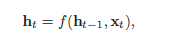其中

h

0

=

0

，

f

(

⋅

)

h_0 = 0，f(·)

为一个非线性函数，也可以是一个前馈网络。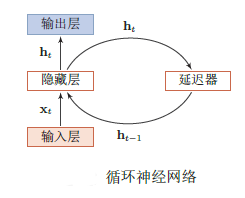简单循环网络SRN
SRN是一个非常简单的循环神经网络，只有一个隐藏层的神经网络。 在一个两层的前馈神经网络中，连接存在相邻的层与层之间，隐藏层的节点之间是无连接的。而简单循环网络增加了从隐藏层到隐藏层的反馈连接。 假设在时刻

t

t

时，网络的输入为

x

t

x_t

，隐藏层状态（即隐藏层神经元活性值）为

h

t

h_t

不仅和当前时刻的输入

x

t

x_t

相关，也和上一个时刻的隐藏层状态

h

t

−

1

h_{t_-1}

相关。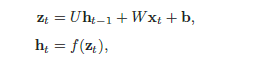其中

z

t

z_t

为隐藏层的净输入，

f

(

⋅

)

f(·)

是非线性激活函数，通常为Logistic函数或Tanh函数，

U

U

为状态-状态权重矩阵，

W

W

为状态-输入权重矩阵，

b

b

为偏置。上述公式经常直接写为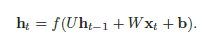如果我们把每个时刻的状态都看作是前馈神经网络的一层的话，循环神经网络可以看作是在时间维度上权值共享的神经网络。按时间展开的循环神经网络如下：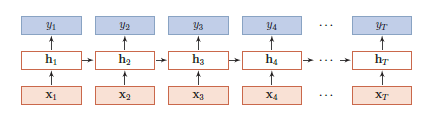参数学习
循环神经网络的参数可以通过梯度下降方法来进行学习。 以随机梯度下降为例，给定一个训练样本

(

x

,

y

)

(x, y)

，其中

x

1

:

T

=

(

x

1

,

⋅

⋅

⋅

,

x

T

)

x_1:T = (x_1, · · · , x_T )

为长度是

T

T

的输入序列，

y

1

:

T

=

(

y

1

,

⋅

⋅

⋅

,

y

T

)

y_1:T = (y_1, · · · , y_T )

是长度为

T

T

的标签序列。即在每个时刻

t

t

，都有一个监督信息

y

t

y_t

，我们定义时刻t 的损失函数为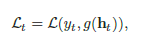其中

g

(

h

t

)

g(h_t)

为第

t

t

时刻的输出，

L

L

为可微分的损失函数，比如交叉熵。那么整个序列上损失函数为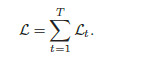整个序列的损失函数

L

L

关于参数

U

U

的梯度为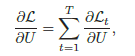即每个时刻损失

L

t

L_t

对参数

U

U

的偏导数之和。 循环神经网络中存在一个递归调用的函数f(·)，因此其计算参数梯度的方式和前馈神经网络不太相同。在循环神经网络中主要有两种计算梯度的方式：随时间反向传播（BPTT）和实时循环学习（RTRL）算法。
随时间反向传播算法BPTT
随时间反向传播（Backpropagation Through Time，BPTT）算法的主要思想是通过类似前馈神经网络的错误反向传播算法来进行计算梯度。 BPTT算法将循环神经网络看作是一个展开的多层前馈网络，其中“每一层”对应循环网络中的“每个时刻”。这样，循环神经网络就可以按照前馈网络中的反向传播算法进行计算参数梯度。在“展开”的前馈网络中，所有层的参数是共享的，因此参数的真实梯度是将所有“展开层”的参数梯度之和。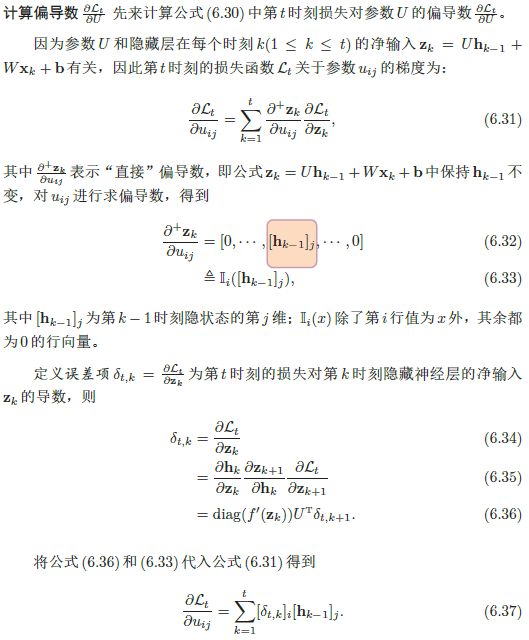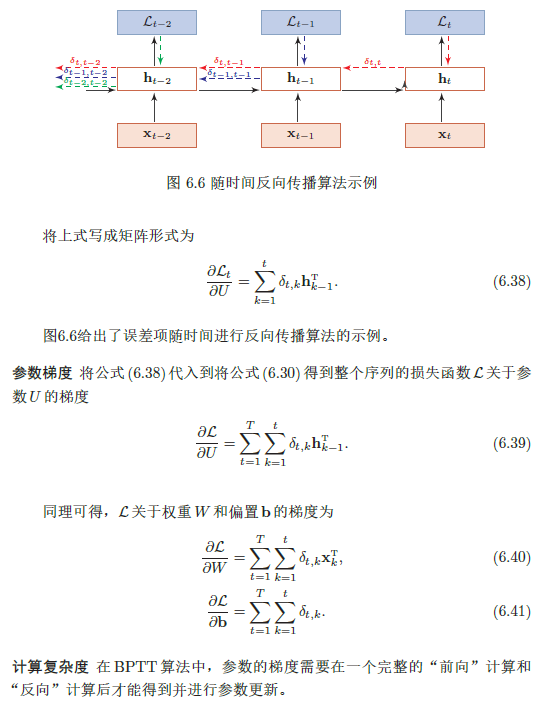实时循环学习算法RTRL
与反向传播的BPTT算法不同的是，实时循环学习（Real-Time RecurrentLearning，RTRL）是通过前向传播的方式来计算梯度。 假设循环神经网络中第

t

+

1

t+1

时刻的状态

h

t

+

1

h_{t+1}

为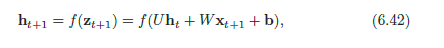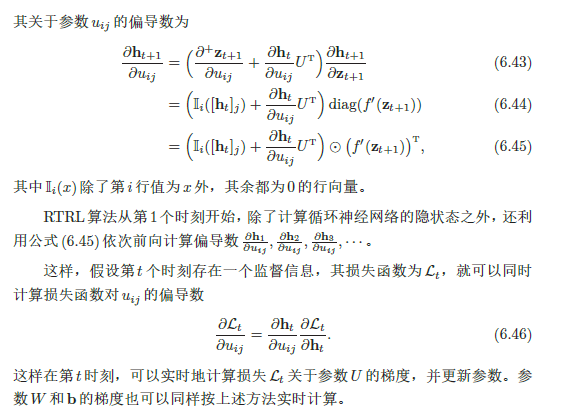两种算法比较 RTRL算法和BPTT算法都是基于梯度下降的算法，分别通过前向模式和反向模式应用链式法则来计算梯度。在循环神经网络中，一般网络输出维度远低于输入维度，因此BPTT算法的计算量会更小，但是BPTT算法需要保存所有时刻的中间梯度，空间复杂度较高。RTRL算法不需要梯度回传，因此非常适合用于需要在线学习或无限序列的任务中。
长期依赖问题
循环神经网络在学习过程中的主要问题是长期依赖问题。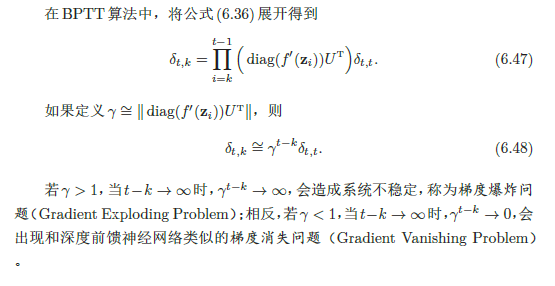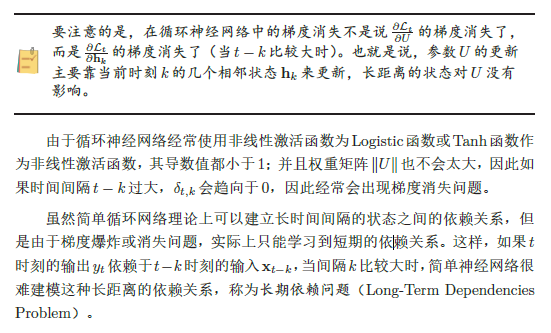改进方案：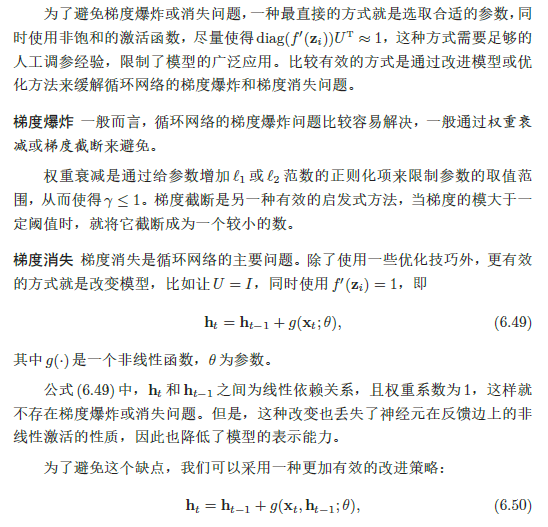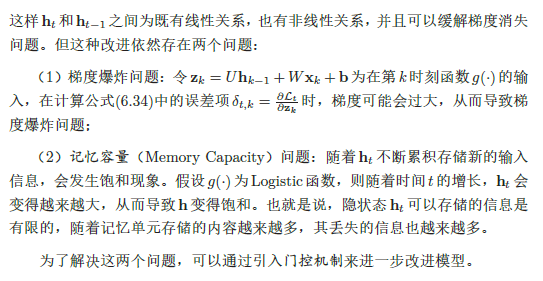基于门控的循环神经网络
为了改善循环神经网络的长期依赖问题，一种非常好的解决方案是在公式(6.50)的基础上，引入门控机制来控制信息的累积速度，包括有选择地加入新的信息，并有选择地遗忘之前累积的信息。这一类网络可以称为基于门控的循环神经网络（Gated RNN）。本节中，主要介绍两种基于门控的循环神经网络：长短期记忆网络和门控循环单元网络。
长短期记忆网络LSTM
LSTM是循环神经网络的一个变体，可以有效地解决简单神经网络的梯度爆炸或消失问题。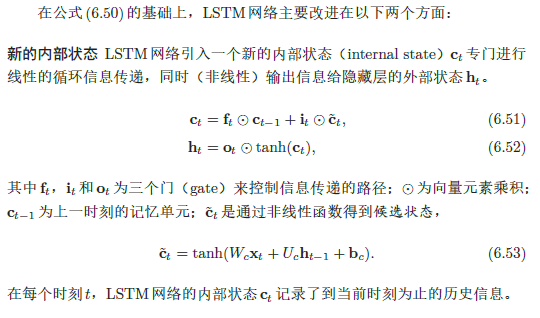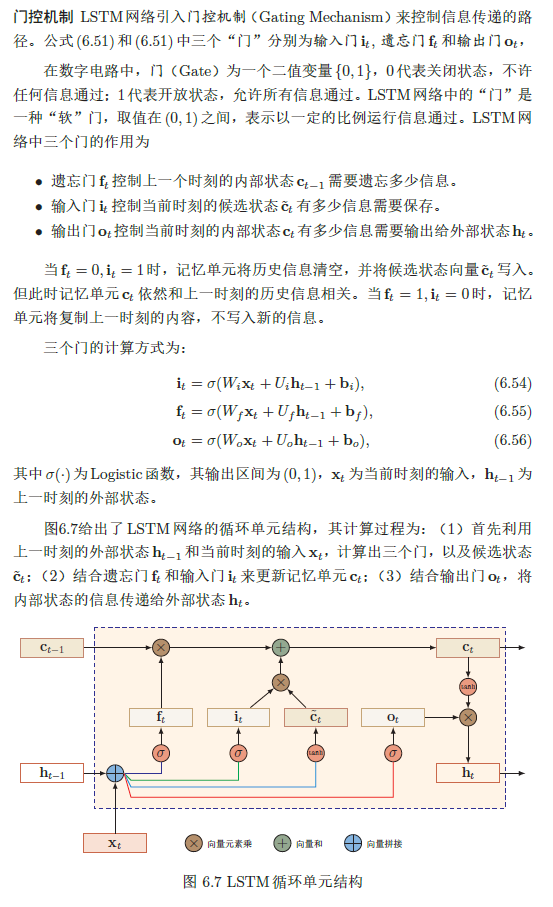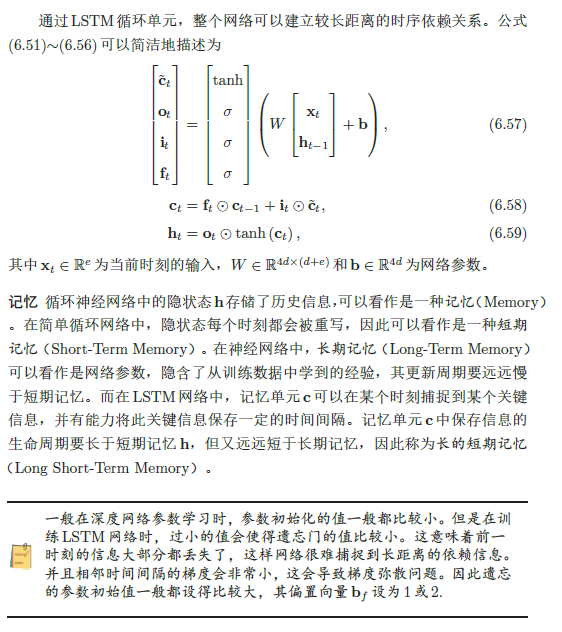门控循环单元网络GRU
门控循环单元（Gated Recurrent Unit，GRU）网络是一种比LSTM网络更加简单的循环神经网络。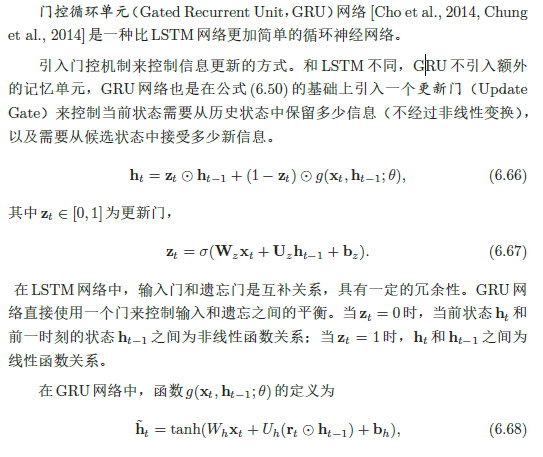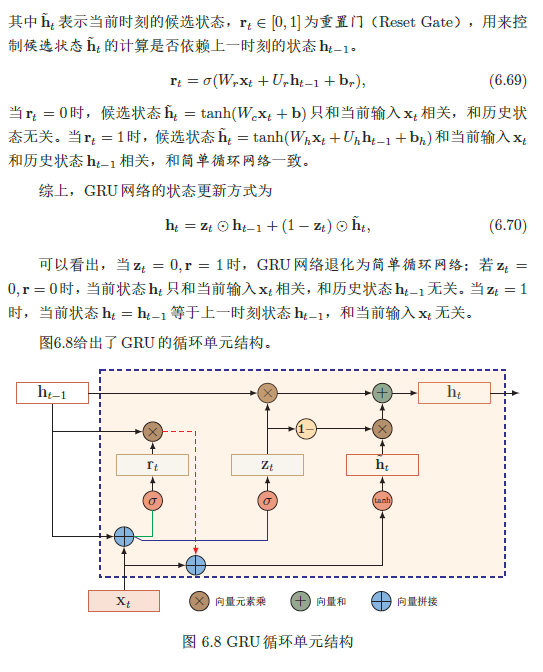RNN文本分类
用于文本分类的单向RNN结构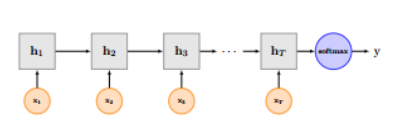引入双向LSTM进行分类,网络框架是:embedding layer—>Bi-LSTM layer—>concat output—>FC layer —> softmax层 BiLSTM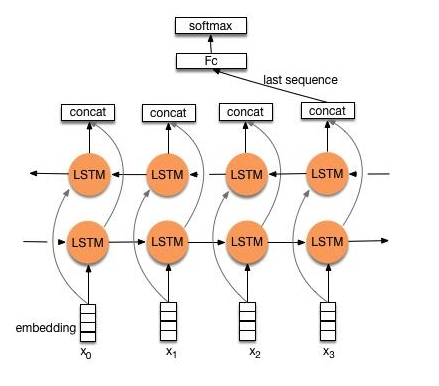BiLSTM multilayers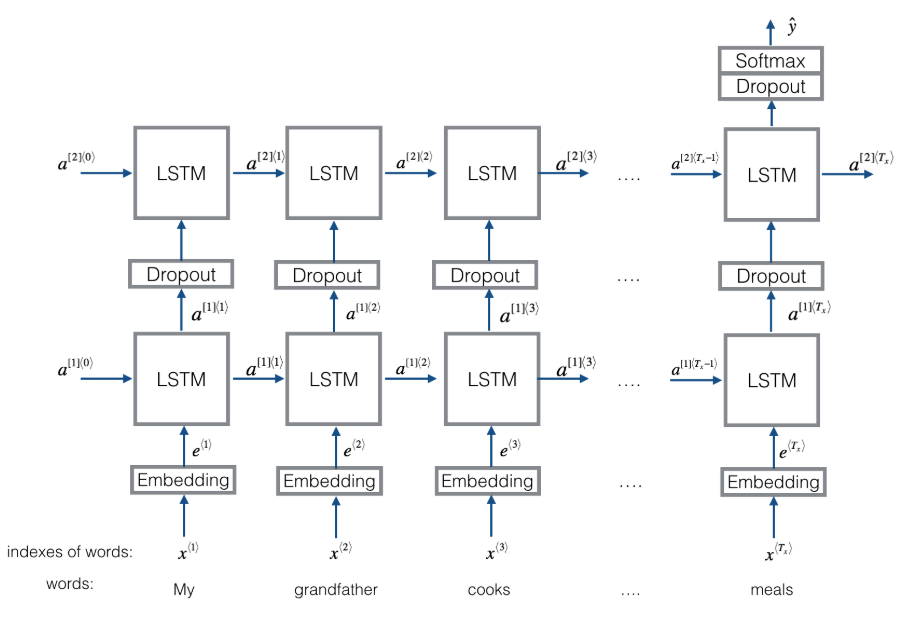Recurrent Convolutional Neural Networks(RCNN)
RCNN网络结构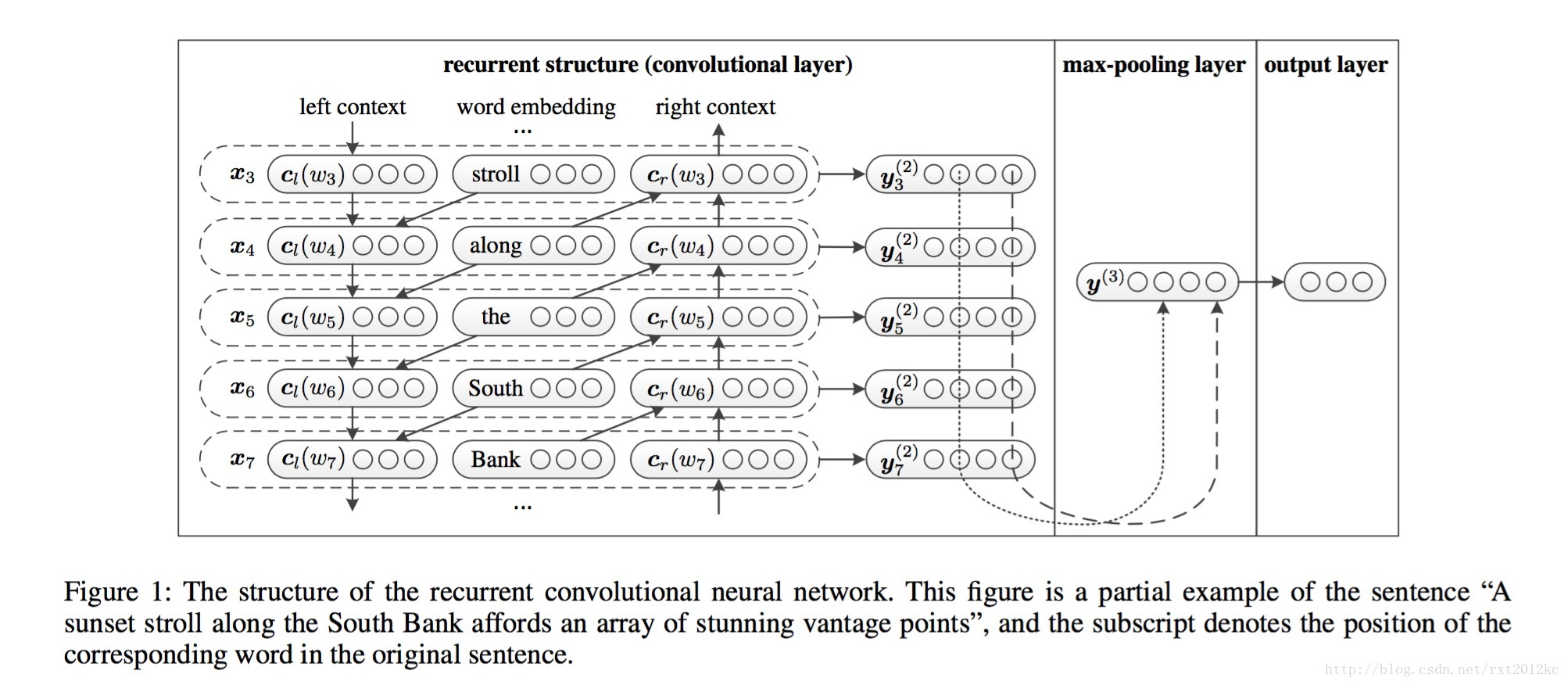首先，构造CNN的卷积层，卷积层的本质是一个BiRNN模型，通过正向和反向循环来构造一个单词的下文和上文，如下式：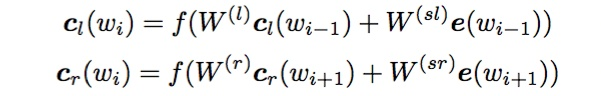得到单词的上下文表示之后，用拼接的方式来表示这个单词，如下式：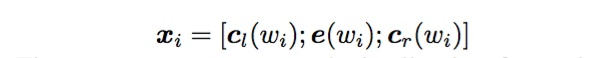将该词向量放入一个单层神经网络中，得到所谓的潜语义向量（latent semantic vector），这里卷积层的计算结束了，时间复杂度仍是O(n)。接下来进行池化层（max-pooling），即将刚刚得到的所有单词的潜语义向量中每个维度上最大的值选出组成一个新的向量，这里采用max-pooling可以将向量中最大的特征提取出来，从而获取到整个文本的信息。池化过程时间复杂度也是O(n)，所以整个模型的时间复杂度是O(n)。得到文本特征向量之后，进行分类。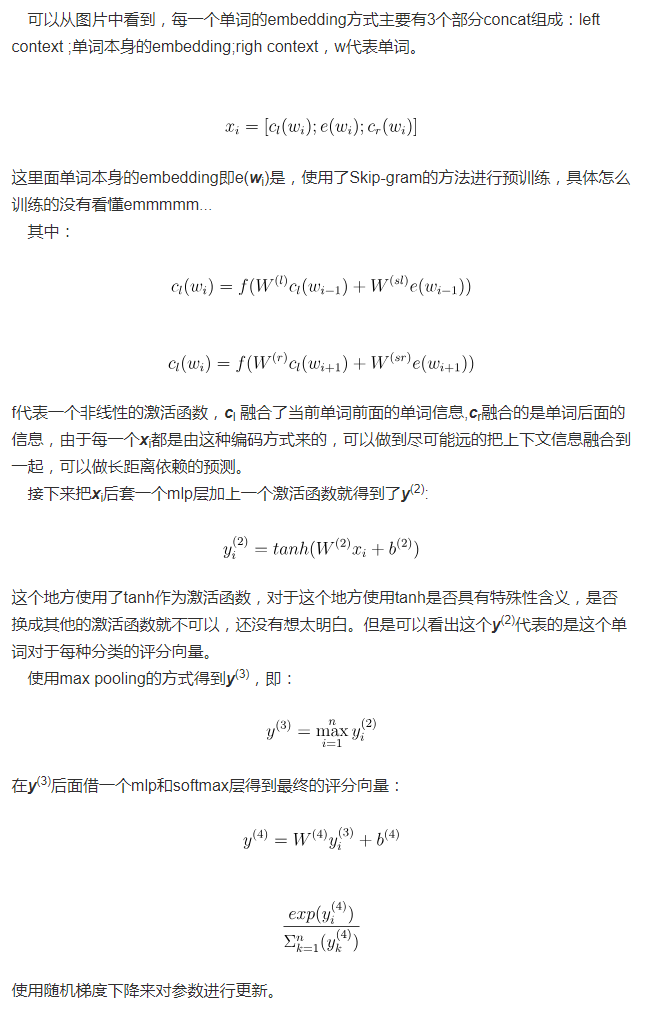RCNN灵活地结合RNN和CNN构造了新的模型，利用了两种模型的优点，提升了文本分类的性能。这也提供了一种研究思路，因为每一种model都有其鲜明的优点和无法回避的缺点，如何利用别的model的优点来弥补自身model的缺点，是改进model的一种重要思路。

Text-RNN文本分类
Text-RNN.py
RCNN文本分类
RCNN.py
参考资料
TextRNN用于文本分类 text-classification-cnn-rnn Recurrent Convolutional Neural Networks for Text Classification 论文随便读:Recurrent Convolutional Neural Networks forText Classification RCNN文本分类与tensorflow实现 神经网络与深度学习
展开全文• TensorFlow2循环神经网络
TensorFlow2循环神经网络

文章目录
TensorFlow2循环神经网络简介序列数据循环神经网络循环神经网络单元LSTM（长短时记忆单元）GRURNN文本情感分类实战LSTM文本情感分类实战补充说明

简介
循环神经网络（recurrent neural network）由于其对时序数据强大的处理能力，被广泛应用于自然语言处理（NLP）领域。近些年，随着各个研究领域的融合趋势，RNN为基础的一些结构如LSTM也被使用在计算机视觉中的任务中。
序列数据
序列信号（Sequence）通常通过序列嵌入（Sequence embedding）来表达，格式为[b, seq_len, feature_len]。用NLP的观点理解，其中b批尺寸（batch size），seq_len为句子单词数目，feature_len为表示一个单词的向量维度（如300维的词向量）。
如果将图片理解为序列数据，[b, 28, 28]则表示每一行是一次采样的结果。当然，RNN在图片上有时有着不错的效果，但是很多时候效果很一般，这是因为RNN本身是用来处理时序数据的，并不擅长处理图片这类数据。
onehot编码
对共有1000个单词的词表，每个单词用一个1000维的向量表示，其中属于这个单词的位置置1，其余位置为0.这是一种及其暴力简单的编码方式，不过有着不少的缺点：无法衡量不同单词之间的距离（因为任意两个单词之间的距离是相同的）、所占存储空间大（稀疏高维矩阵）。 word embedding（词嵌入）
词嵌入的产生方法有很多，后来的研究表明比较好的两种方法为Word2Vec和Glove（本质上二者都是上下文预测产生的）。在TF2中，keras模块下的Embedding层用于嵌入的学习。相比于onehot那样的编码方式，embedding为低维密集矩阵，存储方便的同时也保留了语义距离的信息。
循环神经网络
如同卷积神经网络提出的初衷一样，如果使用全连接神经网络处理文本信息（如处理后的词嵌入）势必会构建一个非常复杂的网络，参数量相当庞大。而且这种将每个单词的信息送入全连接网络会忽略自然语言的一个重要问题—上下文相关。
在卷积神经网络中，为了解决过多参数采用了参数共享（weight sharing）方法，同样的，在循环神经网络中，也采用类似的方法，通过同一个线性层提取语义信息。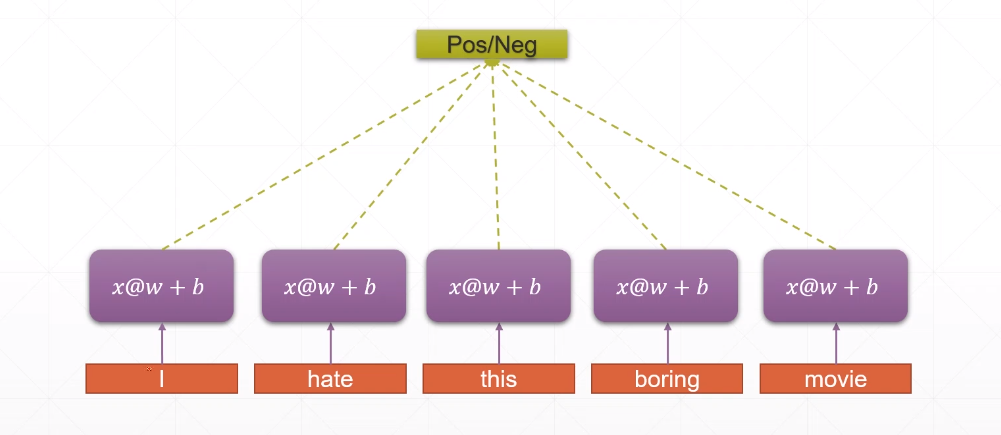但是，自然语言处理不仅仅需要关注每个单词的信息，还需要关注整个句子的信息，因此需要一个记录之前信息的记忆结构h（memory或state），在每个单词处理是都让它参与运算，这样不断累积，最后一个单词后得到的新h(新memory)就记录了整个句子的语义信息。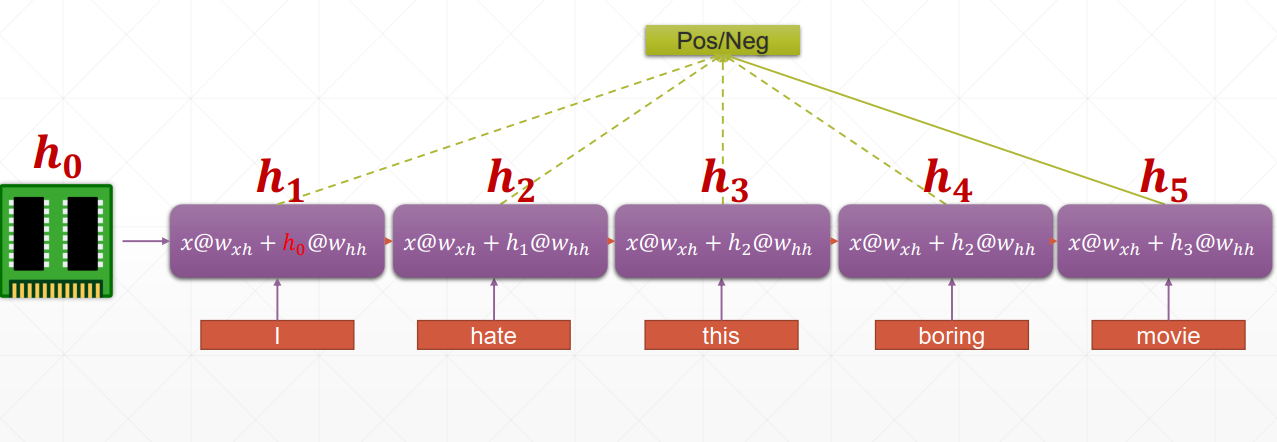上述的模型其实可以折叠，折叠后产生的模型结构如下图，对于不同时间点采样的信息都是以同一种方式提取特征，上一次的信息作为后一次记忆更新的基础，如此反复，形成循环结构，因此成为循环神经网络（RNN）。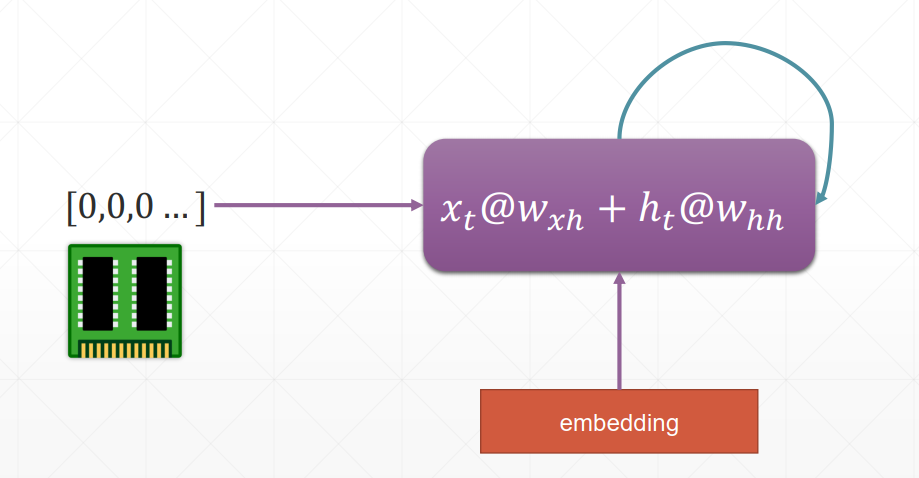数学上表达如下，其中h为中间状态，对于有的模型这个h就是输出，对于有的模型还需要对h进行变换，需要分情况而定。

h

t

=

f

W

(

h

t

−

1

,

x

t

)

h

t

=

tanh

⁡

(

W

h

h

h

t

−

1

+

W

x

h

x

t

)

\begin{array}{c}{h_{t}=f_{W}\left(h_{t-1}, x_{t}\right)} \\ {h_{t}=\tanh \left(W_{h h} h_{t-1}+W_{x h} x_{t}\right)}\end{array}

在探讨完结构后，还有一个关键的问题，就是参数的梯度求解方法，这里依据链式法则拆解并不难求解，其中最为关键的一步求解如下（梯度需要在时间轴上计算，非常复杂，人为难以完成）。

∂

E

t

∂

W

R

=

∑

i

=

0

t

∂

E

t

∂

y

t

∂

y

t

∂

h

t

∂

h

t

∂

h

i

∂

h

i

∂

W

R

\frac{\partial E_{t}}{\partial W_{R}}=\sum_{i=0}^{t} \frac{\partial E_{t}}{\partial y_{t}} \frac{\partial y_{t}}{\partial h_{t}} \frac{\partial h_{t}}{\partial h_{i}} \frac{\partial h_{i}}{\partial W_{R}}

∂

h

k

∂

h

1

=

∏

i

k

diag

⁡

(

f

′

(

W

I

x

i

+

W

R

h

i

−

1

)

)

W

R

\frac{\partial h_{k}}{\partial h_{1}}=\prod_{i}^{k} \operatorname{diag}\left(f^{\prime}\left(W_{I} x_{i}+W_{R} h_{i-1}\right)\right) W_{R}

循环神经网络单元
在TensorFlow2中，对循环神经网络单元的运算进行了简单的封装，通过不断将t时刻的x作为输入送入RNN模块中进行矩阵运算。而第二节所提出的RNN结构是最为简单基础的RNN结构，在keras模块下封装为SimpleRNNCell。该结构进行运算的输出为out, h1 = call(x, h0)，这里的out和h1是同一个东西，之所以返回两次是为了和LSTM等结构做区分。RNNCell也可以堆叠以获取更高层的语义信息。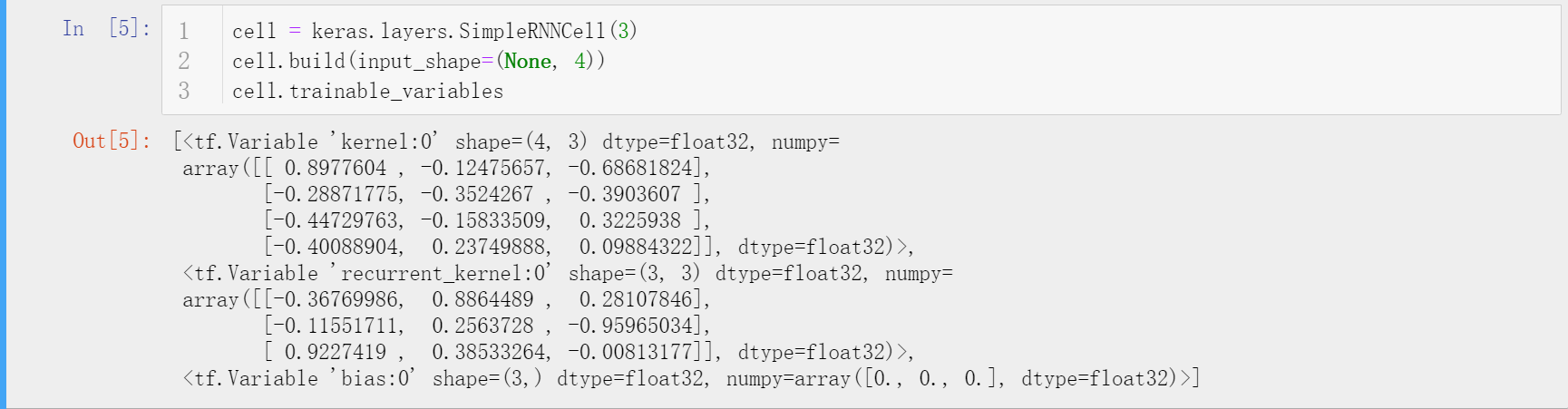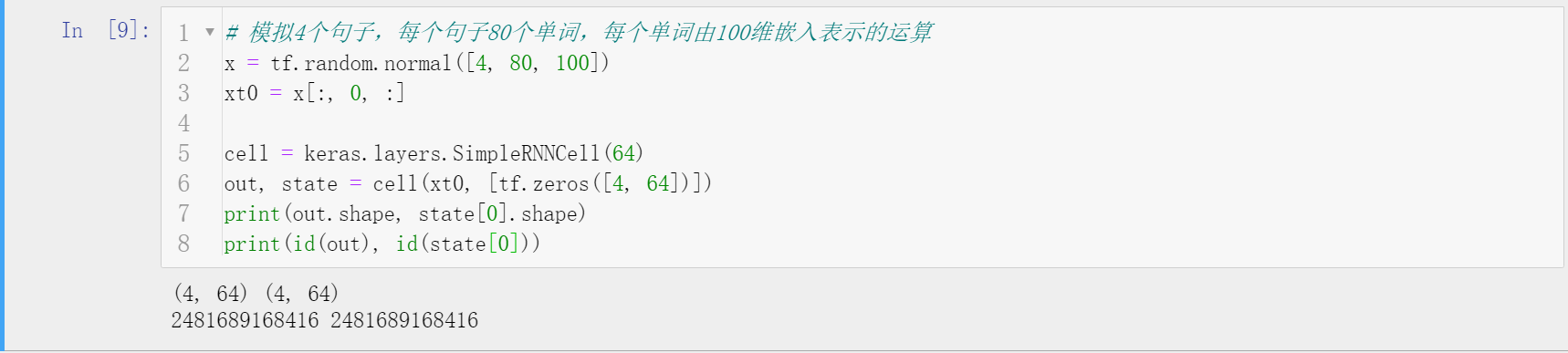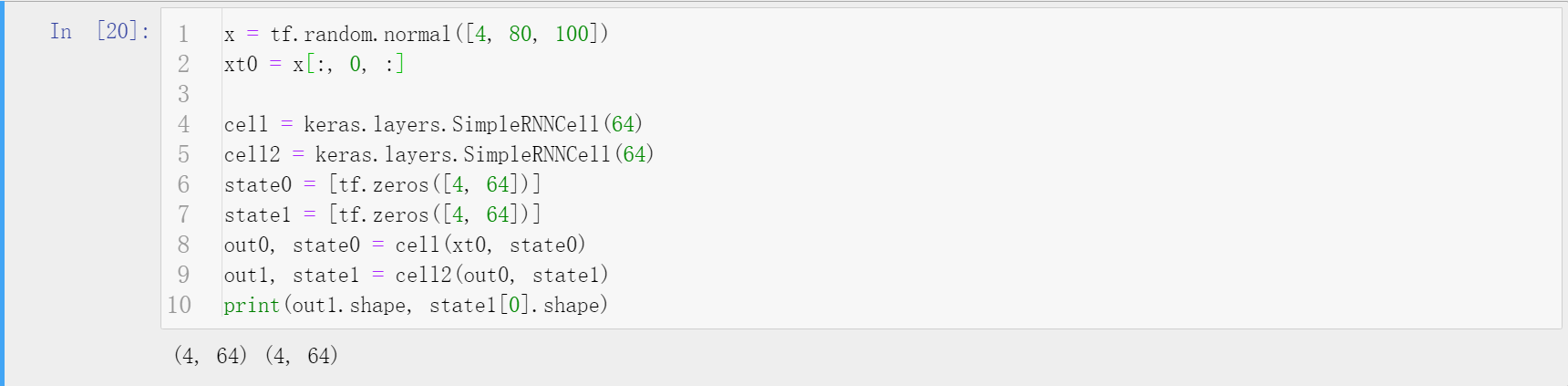实际训练过程中，以文本信息为演示场景，共有两种方法，一种是底层控制（较为灵活，写起来繁琐），一种借助keras的api堆叠SimpleRNN（较为死板，写起来简单）。两种方式的输出都是第二层RNN后t=80时的状态（针对本例）。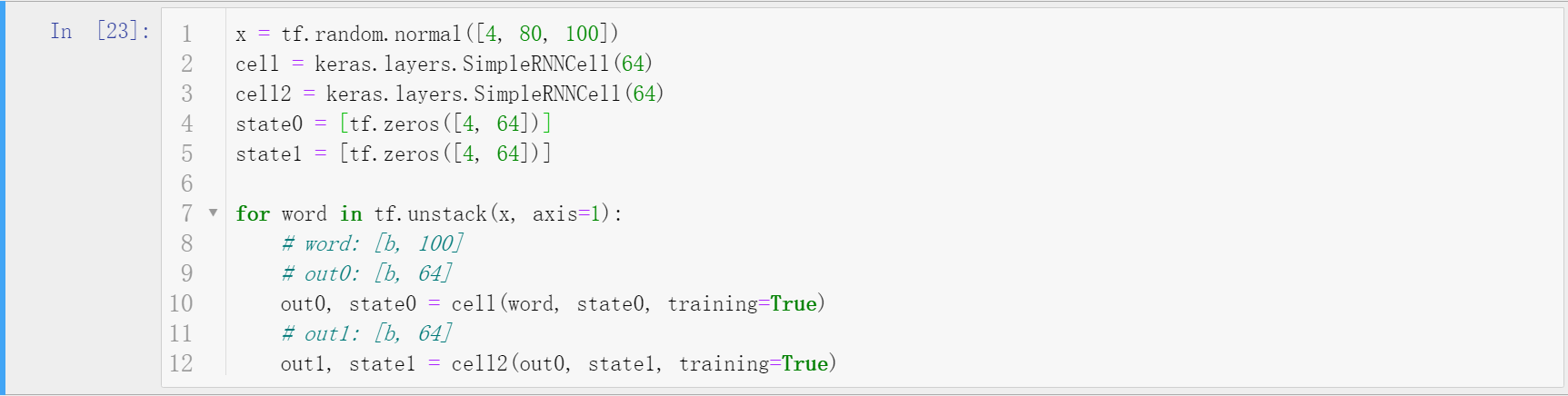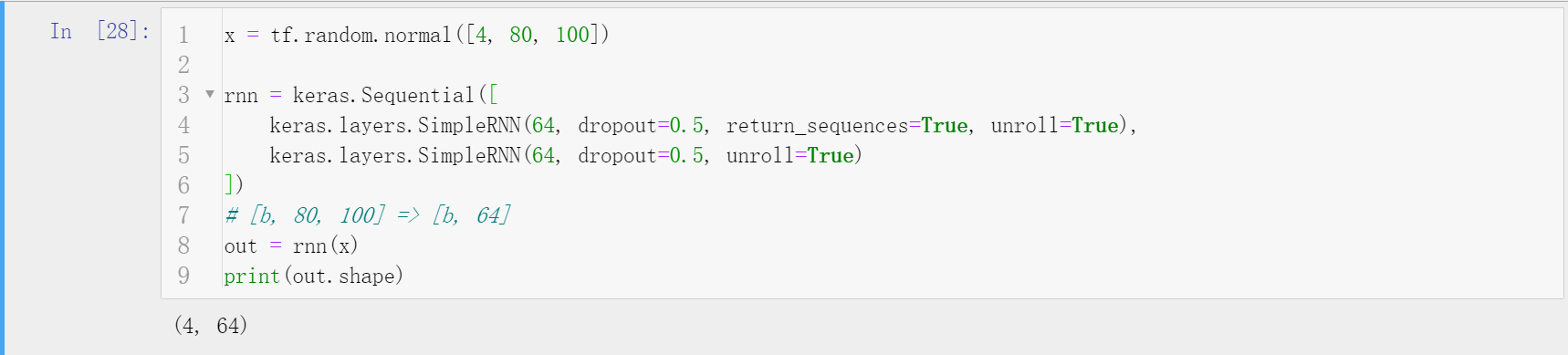LSTM（长短时记忆单元）
目前，主流的模型中很少使用基本的RNN，而是使用LSTM和GRU（减少了LSTM的一个门，在很多情况下效果更好）。
GRU
简化了LSTM，设计了重置门和更新门两个门。
RNN文本情感分类实战
这里通过两种方式构建RNN实现文本情感分类（二分类），数据集使用IMDB电影评论数据集。第一种方法手工实现前向运算，使用SimpleRNN。第二种方法，通过简单的keras接口实现复杂的网络结构堆叠。其代码如下。
"""
Author: Zhou Chen
Date: 2019/11/3
Desc: 底层构建单层RNN实现文本情感分类
"""
import os
import tensorflow as tf
import numpy as np
from tensorflow import keras
from tensorflow.keras import layers

os.environ['TF_CPP_MIN_LOG_LEVEL'] = '2'

total_words = 10000
max_sentence_len = 80  # 每个句子限制80个单词
batch_size = 128
embedding_len = 100
# 加载数据
(x_train, y_train), (x_test, y_test) = keras.datasets.imdb.load_data(num_words=total_words)  # 选取常见的10000个单词

db_train = tf.data.Dataset.from_tensor_slices((x_train, y_train))
db_train = db_train.shuffle(1000).batch(batch_size, drop_remainder=True)
db_test = tf.data.Dataset.from_tensor_slices((x_test, y_test))
db_test = db_test.shuffle(1000).batch(batch_size, drop_remainder=True)
print('x_train shape', x_train.shape, tf.reduce_max(y_train), tf.reduce_min(y_train))
print('x_test shape', x_test.shape)

class MyRNN(keras.Model):

def __init__(self, units):
super(MyRNN, self).__init__()

# [b, 64]
self.state0 = [tf.zeros([batch_size, units])]
self.state1 = [tf.zeros([batch_size, units])]

# transform text to embedding representation
# [b, 80] => [b, 80, 100]
self.embedding = layers.Embedding(total_words, embedding_len, input_length=max_sentence_len)

# [b, 80, 100] , h_dim: 64
# RNN: cell1 ,cell2, cell3
# SimpleRNN
self.rnn_cell0 = layers.SimpleRNNCell(units, dropout=0.5)
self.rnn_cell1 = layers.SimpleRNNCell(units, dropout=0.5)

# fc, [b, 80, 100] => [b, 64] => [b, 1]
self.outlayer = layers.Dense(1)

def call(self, inputs, training=None):
# [b, 80]
x = inputs
# embedding: [b, 80] => [b, 80, 100]
x = self.embedding(x)
# rnn cell compute
# [b, 80, 100] => [b, 64]
state0 = self.state0
state1 = self.state1
for word in tf.unstack(x, axis=1):  # word: [b, 100]
# h1 = x*wxh+h0*whh
# out0: [b, 64]
out0, state0 = self.rnn_cell0(word, state0, training)
# out1: [b, 64]
out1, state1 = self.rnn_cell1(out0, state1, training)

# out: [b, 64] => [b, 1]
x = self.outlayer(out1)
# p(y is pos|x)
prob = tf.sigmoid(x)

return prob

def main():
units = 64
epochs = 4

model = MyRNN(units)
metrics=['accuracy'], experimental_run_tf_function=False)
model.fit(db_train, epochs=epochs, validation_data=db_test)

model.evaluate(db_test)

if __name__ == '__main__':
main()


"""
Author: Zhou Chen
Date: 2019/11/3
Desc: 通过层堆叠方式构建RNN实现文本情感分类
"""
import os
import tensorflow as tf
import numpy as np
from tensorflow import keras
from tensorflow.keras import layers
os.environ['TF_CPP_MIN_LOG_LEVEL'] = '2'

batchsz = 128
total_words = 10000
max_review_len = 80
embedding_len = 100
(x_train, y_train), (x_test, y_test) = keras.datasets.imdb.load_data(num_words=total_words)
# x_train:[b, 80]
# x_test: [b, 80]

db_train = tf.data.Dataset.from_tensor_slices((x_train, y_train))
db_train = db_train.shuffle(1000).batch(batchsz, drop_remainder=True)
db_test = tf.data.Dataset.from_tensor_slices((x_test, y_test))
db_test = db_test.batch(batchsz, drop_remainder=True)
print('x_train shape:', x_train.shape, tf.reduce_max(y_train), tf.reduce_min(y_train))
print('x_test shape:', x_test.shape)

class MyRNN(keras.Model):

def __init__(self, units):
super(MyRNN, self).__init__()

# 生成嵌入的表示
# [b, 80] => [b, 80, 100]
self.embedding = layers.Embedding(total_words, embedding_len,
input_length=max_review_len)

# [b, 80, 100] , h_dim: 64
self.rnn = keras.Sequential([
layers.SimpleRNN(units, dropout=0.5, return_sequences=True, unroll=True),
layers.SimpleRNN(units, dropout=0.5, unroll=True)
])

# fc, [b, 80, 100] => [b, 64] => [b, 1]
self.outlayer = layers.Dense(1)

def call(self, inputs, training=None):
# [b, 80]
x = inputs
# embedding: [b, 80] => [b, 80, 100]
x = self.embedding(x)
# rnn cell compute
# x: [b, 80, 100] => [b, 64]
x = self.rnn(x, training=training)

# out: [b, 64] => [b, 1]
x = self.outlayer(x)
# p(y is pos|x)
prob = tf.sigmoid(x)

return prob

def main():
units = 64
epochs = 4

model = MyRNN(units)
# model.build(input_shape=(4,80))
# model.summary()
loss=tf.losses.BinaryCrossentropy(),
metrics=['accuracy'])
model.fit(db_train, epochs=epochs, validation_data=db_test)

model.evaluate(db_test)

if __name__ == '__main__':
main()


LSTM文本情感分类实战
类似上面的实战任务，利用LSTM实现。 代码类似上面RNN，这里不列举，具体可以在文末给出的Github地址找到。其中也包含GRU实现。
补充说明
本文介绍了RNN、LSTM、GRU在TensorFlow2中的实现，更详细的可以查看官方文档。具体的代码同步至我的Github仓库欢迎star；博客同步至我的个人博客网站，欢迎查看其他文章。如有疏漏，欢迎指正。
展开全文• 双向循环神经网络（Bidirectional RNN） 双向RNN模型（BRNN），可以在序列的某点处不但获取之前的信息，同时还可以获取这个序列点之后的信息，说的炫酷点就是get information from the future。 而标准的RNN ...
目录
双向循环神经网络（Bidirectional RNN）
深层循环神经网络（Deep RNNs）

双向循环神经网络（Bidirectional RNN）
双向RNN模型（BRNN），可以在序列的某点处不但获取之前的信息，同时还可以获取这个序列点之后的信息，说的炫酷点就是get information from the future。
而标准的RNN blocks、GRU units、LSTM units都只是前向的（单向）。
标准的RNN blocks：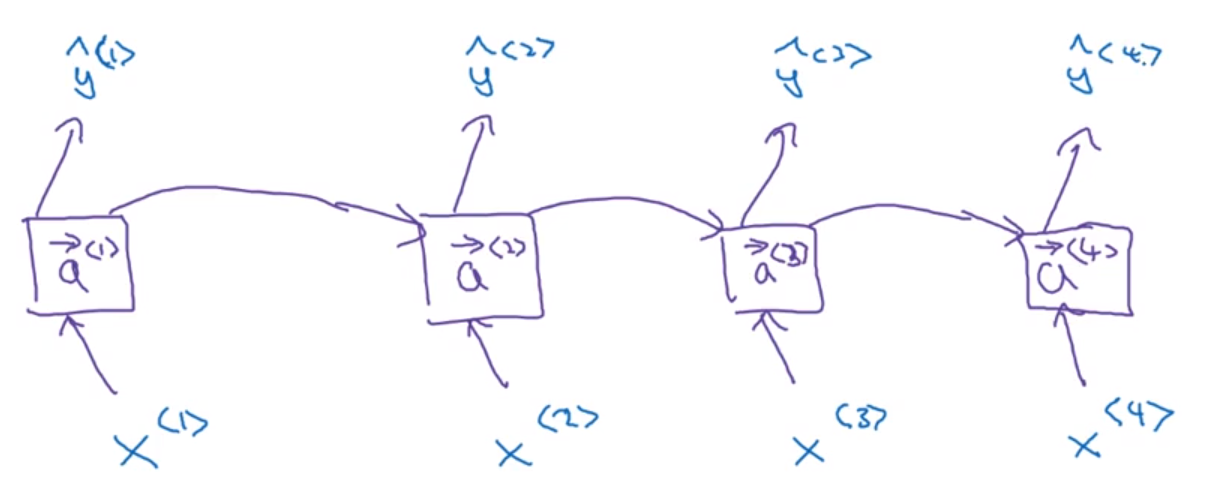双向连接是增加了反向循环层，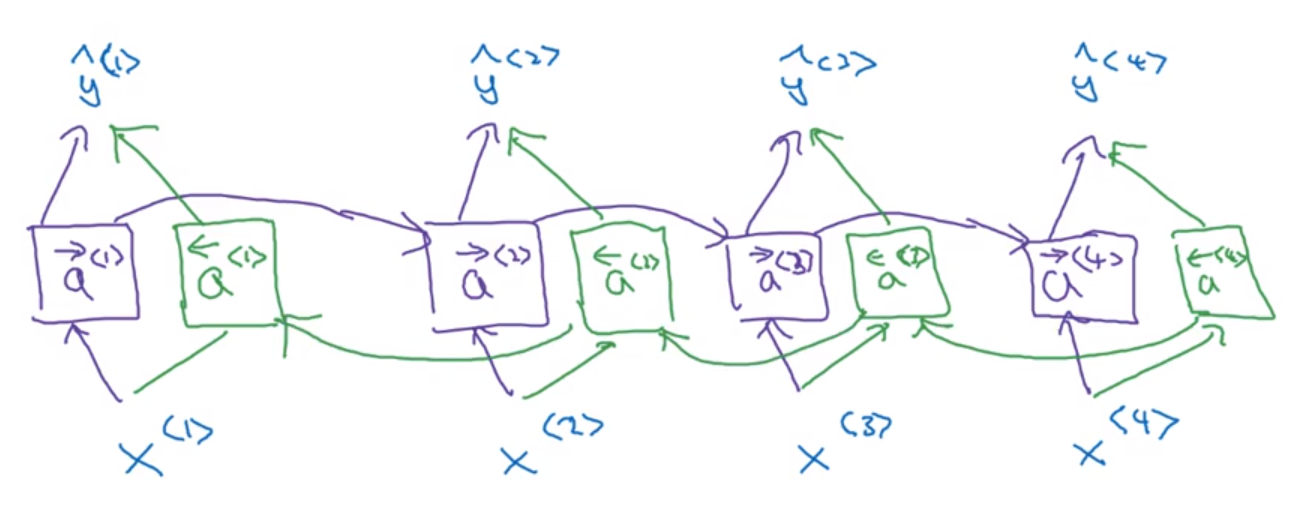（左箭头代表反向连接）
先是从左到右的前向传播计算紫色框内的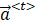，然后再从右到左的前向传播计算绿色框内的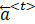，然后计算完所有的激活值后再预测结果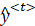。（注意这里的绿色框计算不是反向传播）。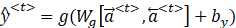例如y<3>可以理解为由前面3个紫色框＋最后1个绿色框决定，这样所有的x<t>的信息都考虑在内了。
这就是双向循环神经网络，并且这些基本单元不仅仅是标准RNN单元，也可以是GRU单元或者LSTM单元。
双向RNN的缺点是需要完整的数据的序列，才能预测任意位置。比如说你要用双向RNN模型构建一个语音识别系统，你需要等待这个人说完，然后获取整个语音表达才能处理这段语音，并进一步做语音识别。

深层循环神经网络（Deep RNNs）
为了学习非常复杂的函数，通常会把RNN的多个层堆叠在一起构建更深的模型。中 <l>表示层数  <t>表示时间步数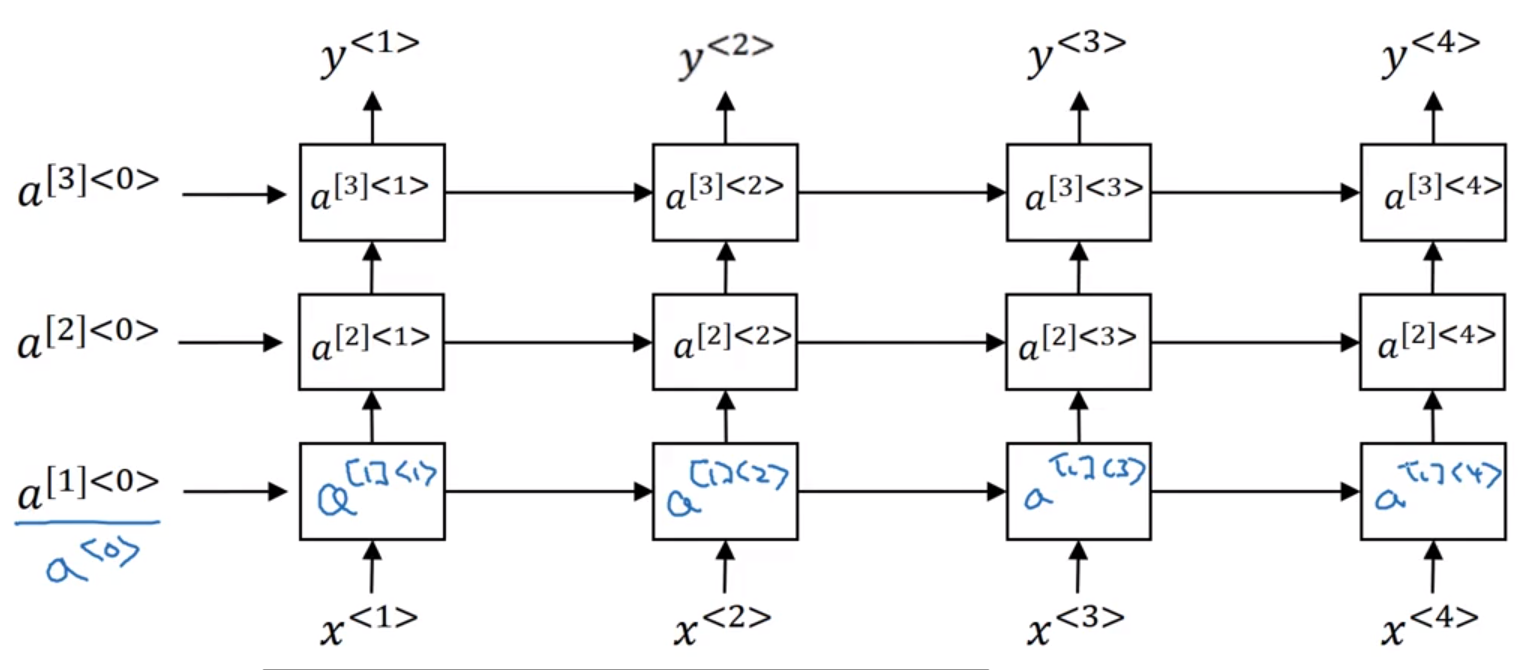以三层的RNN网络为例，GRU和LSTM一样可以堆叠成多层网络。（CNN有100多层很常见，但是RNN一般只有个位数的层数，因为x<t>非常长）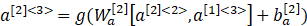，
另外一种加深网络的方式，开头用循环网络，后面加深普通网络如下图蓝色：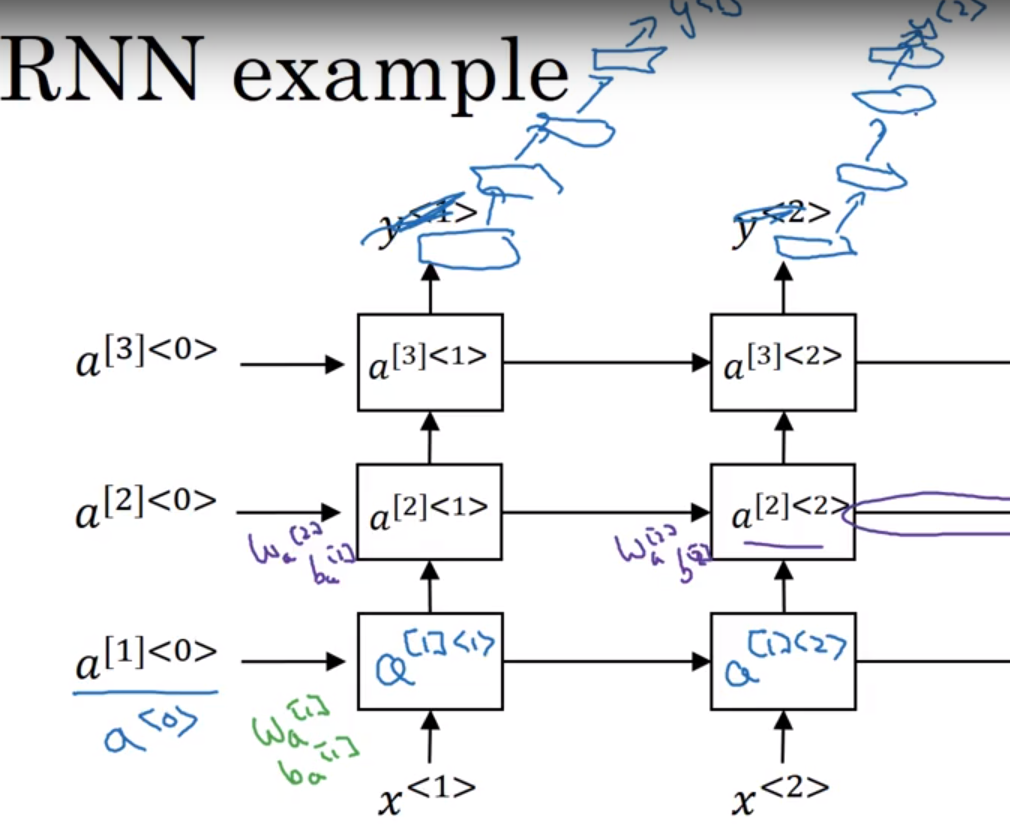话说复杂模型可以构造为 深层双向的LSTM网络。


展开全文深度学习 人工智能
• 本文综合整理常用的神经网络，包括生物神经网络、人工神经网络、卷积神经网络、循环神经网络、生成对抗网络；参考了许多高校的课程、论文、博客和视频等。文章的结构是先进行概念了解，然后结合图片、结构图、一步...
前言
本文综合整理常用的神经网络，包括生物神经网络、人工神经网络、卷积神经网络、循环神经网络、生成对抗网络；参考了许多高校的课程、论文、博客和视频等。文章的结构是先进行概念了解，然后结合图片、结构图、一步一步详细讲解；大家要不看看？ ( •̀ ω •́ )y

一、人工神经网络
简介：人工神经网络 (Artificial Neural Network, ANN)，由人工神经元构成的网络，模拟人类的大脑；它模拟生物过程以反映人脑某些特征的计算结构。
联系：人工神经元模拟生物神经元；人工神经网络模拟人类的大脑，模拟生物神经网络。
特点：人工神经网络是一个并行、分布处理结构，具有学习能力、泛化能力。
功能：联想记忆功能、非线性映射功能、分类与识别功能、知识处理功能。
详细介绍：一篇文章“简单”认识《人工神经网络》(更新版)
目录大纲：

展开全文人工智能 深度学习
• 循环神经网络导读 循环神经网络（Recurrent Neural Network）是一类以序列数据为输入，在序列的演进方向进行递归且所有节点（循环单元）按链式连接的递归神经网络。其中双向循环神经网络（Bidirectional RNN, Bi-...
• 循环神经网络(RNN, Recurrent Neural Networks)介绍   这篇文章很多内容是参考：...  循环神经网络(Recurrent Neural NRNN LSTM
• 文章目录通俗LSTM长短时记忆循环神经网络介绍LSTM图解处理流程流程图解总结说明PyTorch实战参考资料 版权声明：本文为博主原创文章，转载请注明原文出处！ 写作时间：2019-03-02 18:20:11 本文部分图片素材来自...LSTM 深度学习 PyTorch
•RNN LSTM
• 神经网络概述 神经网络是模拟动物神经网络行为特征，进行分布式并行信息处理的算法数学模型。 从x到h到y可以近似看作动物...循环神经网络（RNN） 引入原因 全连接神经网络和卷积神经网络的缺点 全连接神经网络和卷...机器学习
• 文章目录引言序列模型能解决什么问题符号定义循环神经网络通过时间的方向传播不同类型的循环神经网络语言模型和序列生成 引言 本文是吴恩达深度学习第五课：序列模型。本次课程将会学到如何为自然语言、语言和其他...深度学习 长短记忆网络 RNN LSTM
• 文章目录前言一、 序列的表示方法二、循环神经网络模型三，循环神经网络的意义 前言 我们还有很多如：波形，声音，文本等类型的数据需要进行处理，这些波形直接或间接是可以抽象出时间轴的意义。即有先后顺序之分，...深度学习
• 1、循环神经网络（Recurrent Neural Networks） 2、长依赖存在的问题 3、LSTM 网络 4、LSTMs的核心思想 5、一步一步理解LSTM 6、LSTM的变种 7、总结 1、循环神经网络（Recurrent Neural Networks） 人对一个...深度学习 RNN LSTM
• ## 循环神经网络RNN

千次阅读 2017-12-19 17:34:14
循环神经网络(RNN, Recurrent Neural Networks)介绍    这篇文章转载自：http://blog.csdn.net/heyongluoyao8/article/details/48636251   循环神经网络(Recurrent Neural Networks，RNNs)已经在众多自然语言...
• 循环卷积网络（RNN）笔记总结 -------本文仅为学习笔记，不做任何...    常见的循环神经网络包括双向循环神经网络（Bi-RNN）以及长短期记忆网络（LSTM），之后会对LSTM进行详细的介绍，见主页其它博客内容。卷积
• 几种主要的神经网络一、全连接神经网络二、前馈神经网络（Feedforward neural network，FNN）三、卷积神经网络（Convolutional Neural Network，CNN）四、循环神经网络（Recurrent neural network，RNN ） ...人工智能 深度学习
• 循环神经网络RNN 曾经因为新冠肺炎疫情的原因得到了一个超长假期，但是我没有好好珍惜。等到快开学的时候我才追悔莫及，如果上天再给我一次机会我一定会好好学习，天天向上，不睡懒觉，勤勉读书，励精图治，...深度学习
• ## 循环神经网络总结

千次阅读 2019-01-09 22:33:41
循环神经网络(Recurrent Neural Networks ，RNN)是一种输出和模型间有反馈的神经网络。 1. RNN概述 DNN和CNN中，训练样本的输入和输出是比较的确定的。但当训练样本输入是连续的长短不一的序列，如基于时间的序列...
• 人工神经网络介绍参考： https://blog.csdn.net/fengbingchun/article/details/50274471  ... 这里在以上两篇基础上整理介绍循环神经网络： 前馈网络可以分为若干”层”，各层按信号传输先后顺序依次排列...
• 循环神经网络很早就有了，其结构如下图。注意，这里其实只有一个神经网络结构。而不是7个。下图是为了方便表示，一次把7个时间的网络都画在上面。举个翻译的例子，I am hungry 比如在时间1，我们输入了 I，通过神经...
• 循环神经网络（Recurrent Neural Network, RNN）是一种处理时序型输入的神经网络。它被广泛应用在语音识别、机器翻译、人名识别、文本生成等任务上。RNN 存在多种变体，如 LSTM，GRU，BRNN，深层 RNN 等，这些网络...rnn lstm
• 神经网络学习-介绍循环神经网络RNN 初步理解循环神经网络     在文本处理的问题中，最开始是使用的TF-IDF、马尔可夫链、条件随机场等方法，后续有人将BP神经网络使用在文本相关的处理，但是效果...深度学习 机器学习 数据挖掘 自然语言处理
• 但是对于时间等序列预测，比如天气预报，明天是否下雨不仅仅与今天的状态有关，还可能与之前几天甚至几十天的状态有关，因此这里就需要使用循环神经网络。卷积神经网络是在神经网络上加了一层卷积层，同样的道理，...RNN
• 前言前几篇文章介绍了卷积神经网络，但是在另一个大的领域，在文本领域，也可以说是自然语言领域，卷积神经网络效果就不是很好了。这里介绍下循环神经网络。肯定有人会不理解，不是有卷积神经网络吗？...java python 深度学习 算法
• 将神经网络模型训练好之后，在输入层给定一个x，通过网络之后就能够在输出层得到特定的y，那么既然有了这么强大的模型，为什么还需要RNN（循环神经网络）呢？ ** 为什么需要RNN（循环神经网络） ** 他们都只能单独的...深度学习
• ## 自然语言处理之循环神经网络

千次阅读 多人点赞 2019-04-26 15:23:06
循环神经网络RNN,是一类用于处理序列数据的神经网络。就像卷积网络是专门用于处理网格化数据的神经网络，循环神经网络是专门用于处理序列x(1),…,xTx^{(1)},\dots,x^{T}x(1),…,xT的神经网络。正如卷积网络可以...RNN
• 常用的神经网络模型 卷积神经网络（Convolutional ...循环神经网络（Recurrent Neural Network，RNN）。神经网络是一种节点定向连接成环的人工神经网络，这种网络的内部状态可以展示动态时序行为。 LSTM（Long ...
• 本文重点讲述的是一种被称为attention的方法，有的人将其译为“聚焦”，但觉得这种翻译将原文对神经网络拟人化的手法给扔掉了，因此保留了原来的称谓。Attention，顾名思义，就是让神经网络对部分内容引起注意，而...深度学习
• 转自：... 循环神经网络(RNN, Recurrent Neural Networks)介绍    这篇文章很多内容是参考：http://www.wildml.com/2015/09/recurrent-neural-networks-tutorial-part...